# Beat – An Acoustics Inspired DDoS Attack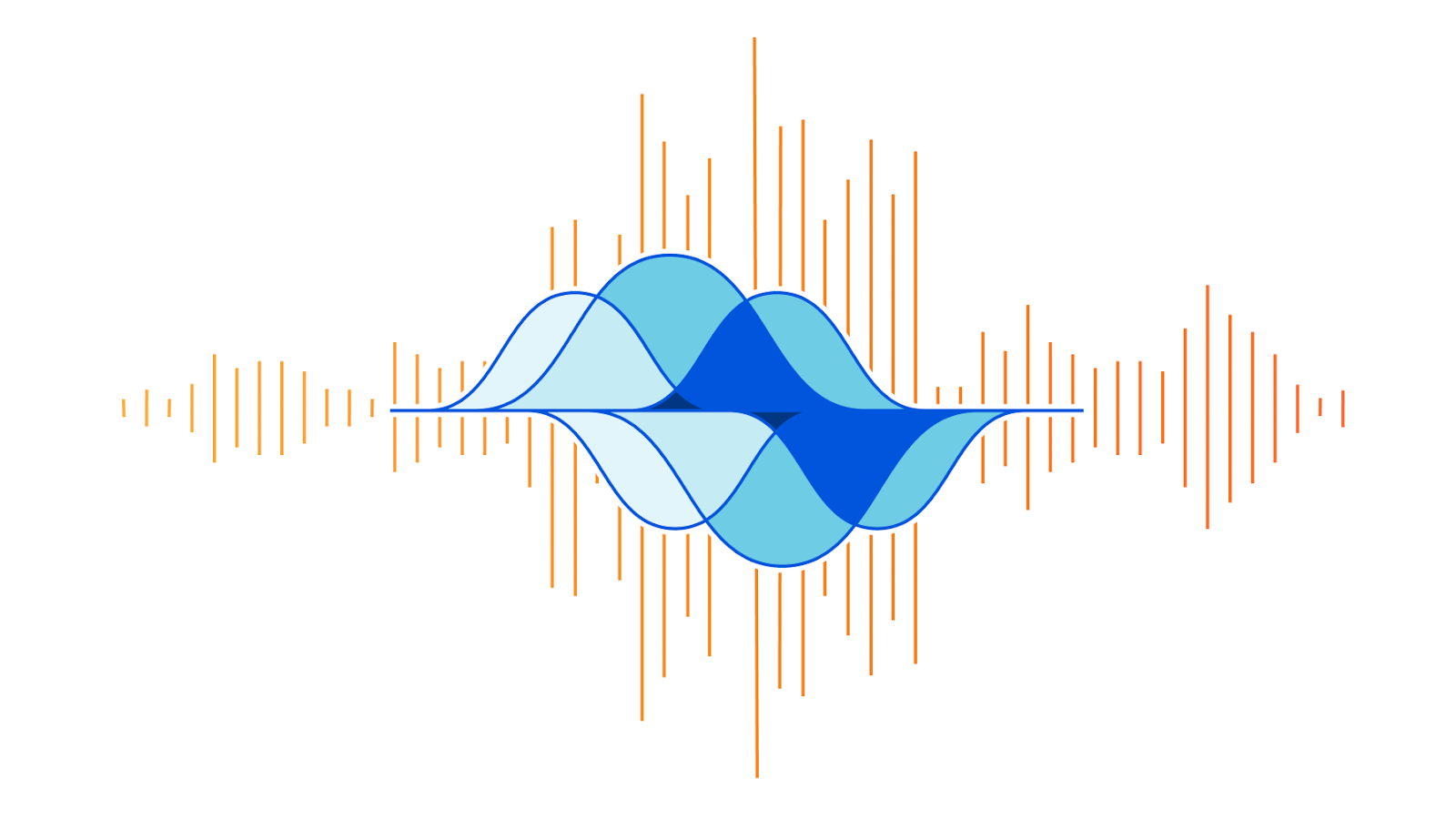On the week of Black Friday, Cloudflare automatically detected and mitigated a unique ACK DDoS attack, which we’ve codenamed “Beat”, that targeted a Magic Transit customer. Usually, when attacks make headlines, it’s because of their size. However, in this case, it’s not the size that is unique but the method that appears to have been borrowed from the world of acoustics.

### Acoustic inspired attack

As can be seen in the graph below, the attack’s packet rate follows a wave-shaped pattern for over 8 hours. It seems as though the attacker was inspired by an acoustics concept called beat. In acoustics, a beat is a term that is used to describe an interference of two different wave frequencies. It is the superposition of the two waves. When the two waves are nearly 180 degrees out of phase, they create the beating phenomenon. When the two waves merge they amplify the sound and when they are out of sync they cancel one another, creating the beating effect.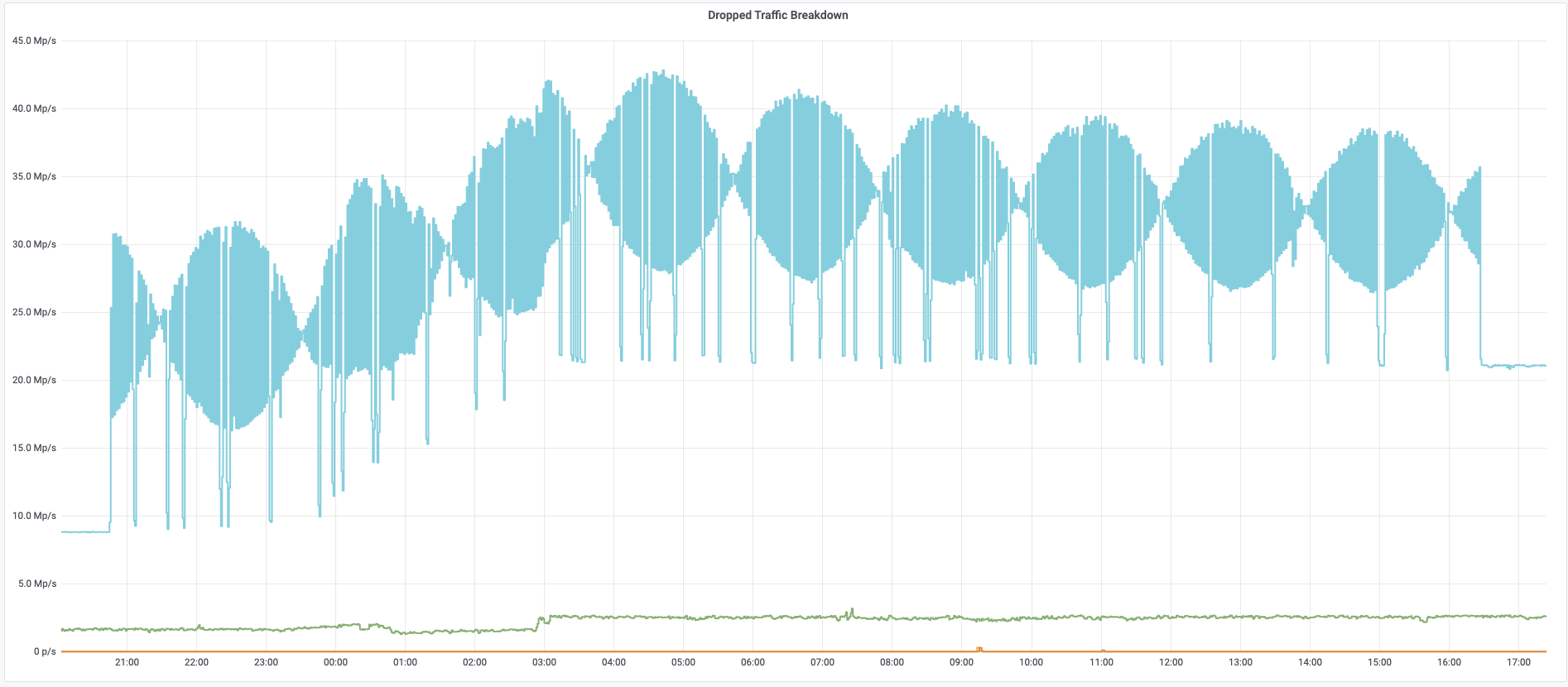Beat DDoS Attack

Acedemo.org has a nice tool where you can create your own beat wave. As you can see in the screenshot below, the two waves in blue and red are out of phase and the purple wave is their superposition, the beat wave.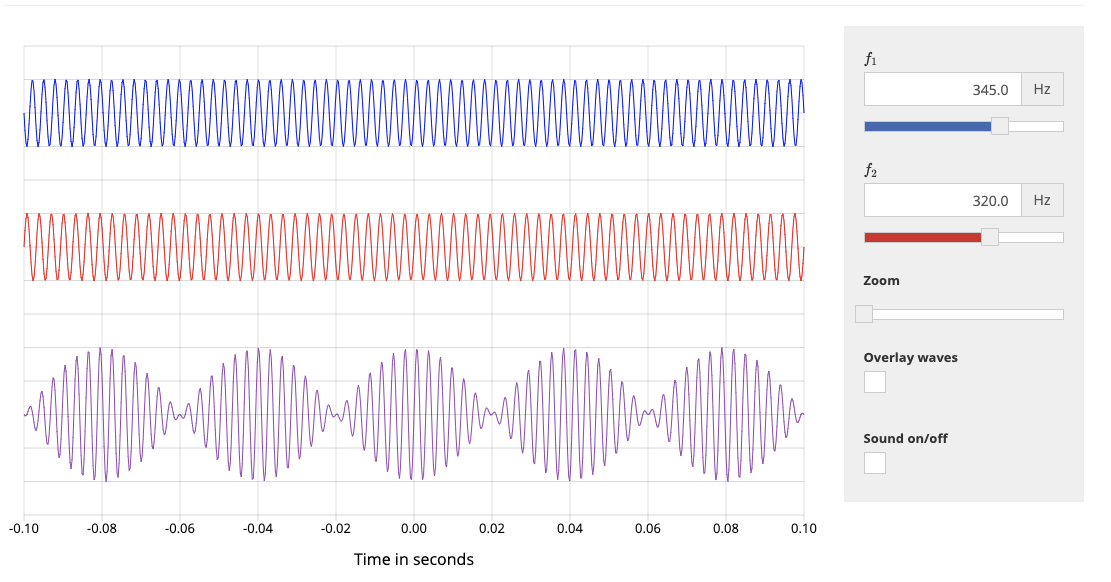Source: https://academo.org/demos/wave-interference-beat-frequency/

### Reverse engineering the attack

It looks like the attacker launched a flood of packets where the rate of the packets is determined by the equation of the beat wave: ybeat=y1+y2. The two equations y1 and y2 represent the two waves.

Each equation is expressed as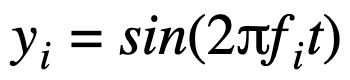where fi is the frequency of each wave and t is time.

Therefore, the packet rate of the attack is determined by manipulation of the equation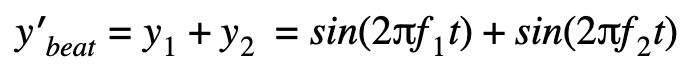to achieve a packet rate that ranges from ~18M to ~42M pps.

To get to the scale of this attack we will need to multiply ybeat by a certain variable a and also add a constant c, giving us ybeat=aybeat+c. Now, it’s been a while since I played around with equations, so I’m only going to try and get an approximation of the equation.

By observing the attack graph, we can guesstimate that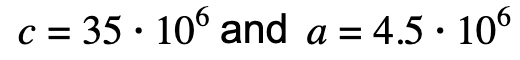by playing around with desmos’s cool graph visualizer tool, if we set f1=0.0000345 and f2=0.00003455 we can generate a graph that resembles the attack graph. Plotting in those variables, we get: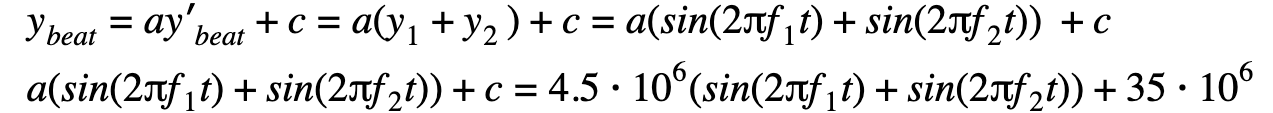Now this formula assumes just one node firing the packets. However, this specific attack was globally distributed, and if we assume that each node, or bot in this botnet, was firing an equal amount of packets at an equal rate, then we can divide the equation by the size of the botnet; the number of bots b. Then the final equation is something in the form of:In the screenshot below, g = f 1. You can view this graph here.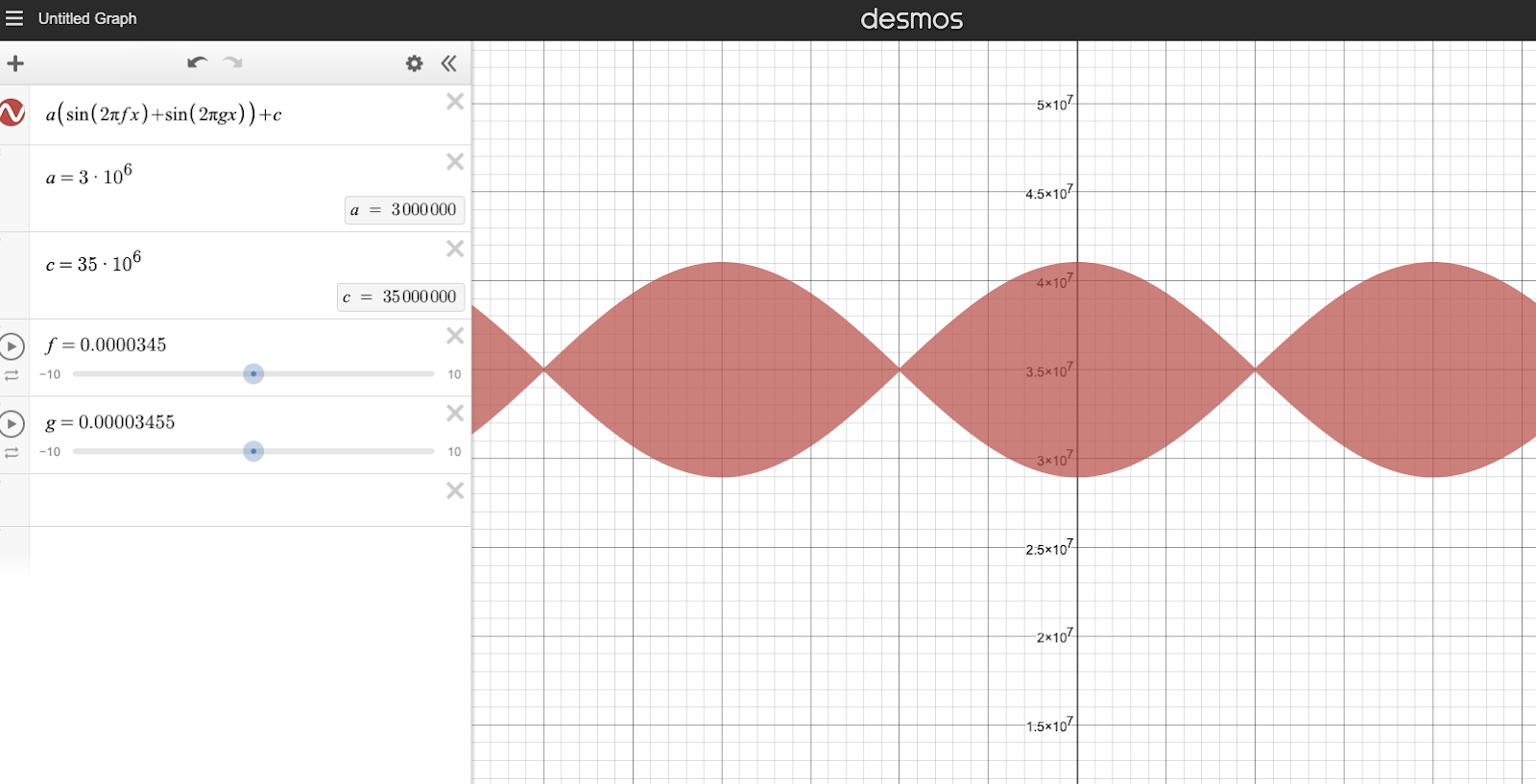### Beating the drum

The attacker may have utilized this method in order to try and overcome our DDoS protection systems (perhaps thinking that the rhythmic rise and fall of the attack would fool our systems). However, about how our DDoS protection systems work here.

Source:: CloudFlare